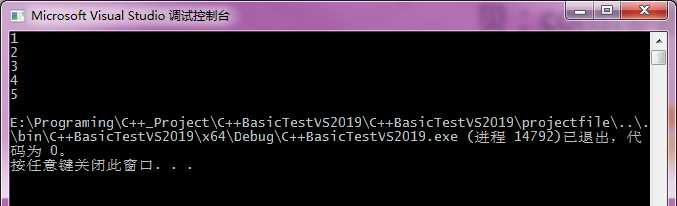# 1 将std::vector中的数值拷贝到数组中

#include <iostream>
#include <vector>
#include <algorithm>

template<typename T>
size_t copy(std::vector<T> const& src, T* dest, size_t N)
{
size_t count = std::min(N, src.size());
std::copy(src.begin(), src.begin() + count, dest);
return count;
}

int main()
{
std::vector<int> testVec = {1,2,3,4,5 };

int* indexArray = new int[testVec.size()];

copy(testVec, indexArray, testVec.size());

for (int i = 0; i < 5; ++i)
{
std::cout << indexArray[i] << std::endl;
}

delete[] indexArray;

return 0;
}